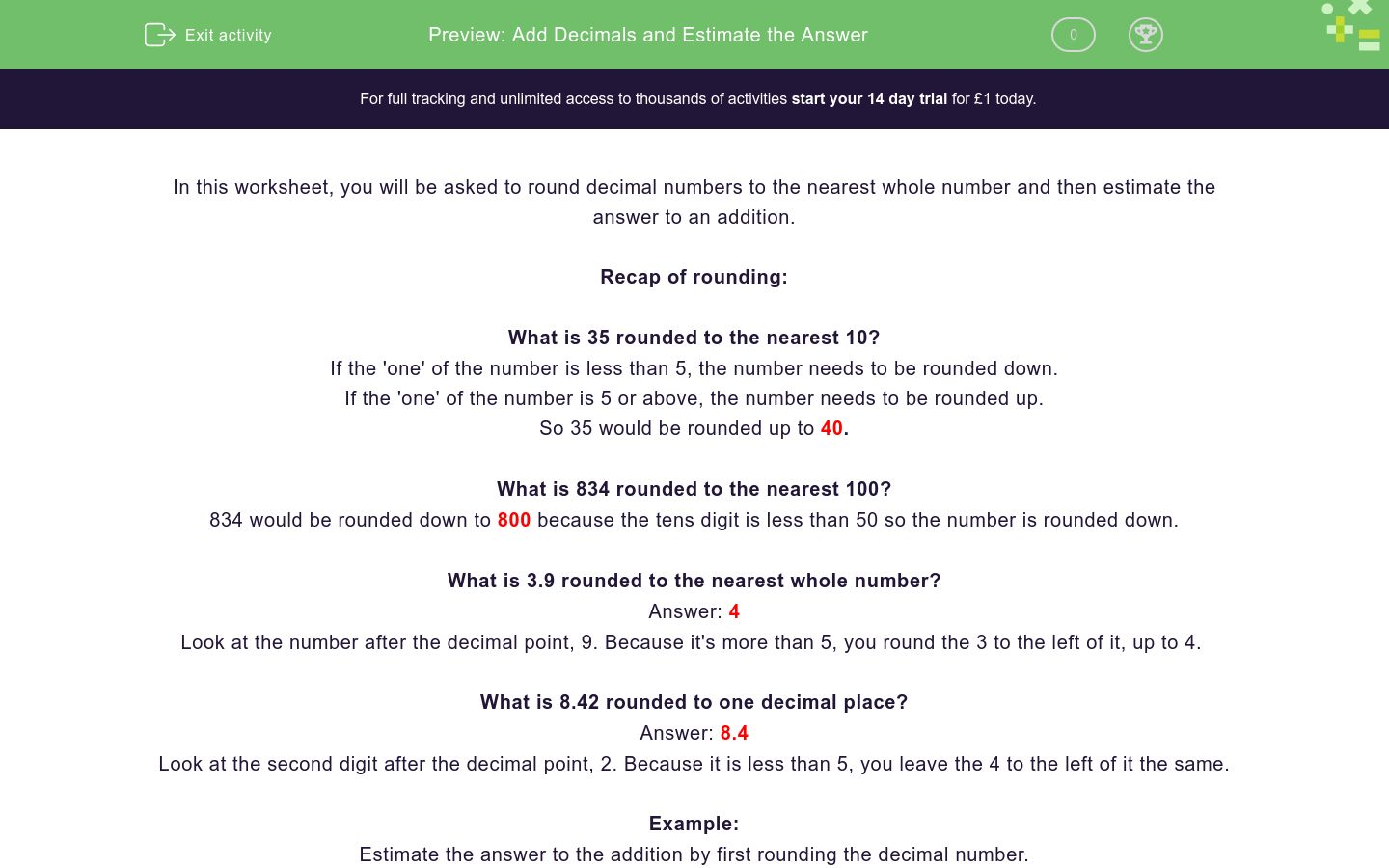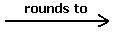### Affordable pricing from £10/month

In this worksheet, students will be asked to estimate the answer to addition calculations by rounding decimal numbers to the nearest whole number.Key stage:  KS 2

Curriculum topic:   Number: Addition, Subtraction, Multiplication and Division

Curriculum subtopic:   Use Estimation to Check Answers

Difficulty level:### QUESTION 1 of 10

In this worksheet, you will be asked to round decimal numbers to the nearest whole number and then estimate the answer to an addition.

Recap of rounding:

What is 35 rounded to the nearest 10?

If the 'one' of the number is less than 5, the number needs to be rounded down.

If the 'one' of the number is 5 or above, the number needs to be rounded up.

So 35 would be rounded up to 40.

What is 834 rounded to the nearest 100?

834 would be rounded down to 800 because the tens digit is less than 50 so the number is rounded down.

What is 3.9 rounded to the nearest whole number?

Look at the number after the decimal point, 9. Because it's more than 5, you round the 3 to the left of it, up to 4.

What is 8.42 rounded to one decimal place?

Look at the second digit after the decimal point, 2. Because it is less than 5, you leave the 4 to the left of it the same.

Example:

Estimate the answer to the addition by first rounding the decimal number.

10 + 1.42 =10 + 1.4 = 11.4

Estimate the answer by rounding the numbers to the nearest 100.

266 + 913A + B = C

Remember to put your answers in the same sequence as the question.

For example, 266 would be A and 913 would be B...

## Column B

B
300
C
900
A
1200

Estimate the answer by rounding the numbers to the nearest 100.

667 + 309A + B = C

Remember to put your answers in the same sequence as the question.

For example, 667 would be A and 309 would be B...

## Column B

C
300
B
1000
A
700

Estimate the answer to the addition by first rounding both decimals to the nearest whole numbers.

43.6 + 19.1A + B = C

Remember to put your answers in the same sequence as the question.

For example, 43.6 would be A and 19.1 would be B...

## Column B

B
19
A
44
C
63

Estimate the answer to the addition by first rounding both numbers to the nearest whole numbers.

82.7 + 14.8?

82 + 14 = 96

83 + 15 = 98

80 + 15 = 95

82.5 + 14.5 = 97

Estimate the answer to the addition by first rounding both numbers to the nearest whole numbers.

Choose the correct calculation, showing the rounded numbers.

112.4 + 26.9?

112 + 27 = 139

113 + 27 = 140

111 + 28 = 139

112.5 + 27 = 139.5

Estimate the answer to the addition by first rounding both decimal numbers to the nearest whole number.

Then choose the correct sequence for the calculation;

126.1 + 127.8?

122 + 130 = 252

125 + 128 = 253

126 + 127 = 253

126 + 128 = 254

Estimate the answer to the addition by rounding the second number to one decimal place.

Then choose the correct sequence for the calculation;

133 + 64.23?

133 + 64 = 197

133 + 64.2 = 19.2

134 + 64.2 = 198.2

133 + 64.5 = 197.5

Estimate the answer to the addition by rounding both numbers to one decimal place.

Then can you complete the blanks below to show the correct sequence?

63.12 + 24.89?

133 + 64 = 197

133 + 64.2 = 19.2

134 + 64.2 = 198.2

133 + 64.5 = 197.5

Estimate the answer to the addition by rounding both numbers to one decimal place.

Then can you complete the blanks below to show the correct sequence?

136.26 + 51.33?

133 + 64 = 197

133 + 64.2 = 19.2

134 + 64.2 = 198.2

133 + 64.5 = 197.5

Estimate the answer to the addition by rounding both decimal numbers to one decimal place.

Then you need to correctly choose the entire calculation, with the answer, in the box.

171.34 + 112.17?

171.3 + 112.2 = 283.5

171 + 112 = 283

172 + 113 = 285

171.4 + 112.1 = 283.5

• Question 1

Estimate the answer by rounding the numbers to the nearest 100.

266 + 913A + B = C

Remember to put your answers in the same sequence as the question.

For example, 266 would be A and 913 would be B...

## Column B

B
900
C
1200
A
300
EDDIE SAYS
So, we have started off by rounding to the nearest 100. 266 rounded up to 300 and 913 rounded down to 900. The calculation we should have is; 300 + 900 = 1200
• Question 2

Estimate the answer by rounding the numbers to the nearest 100.

667 + 309A + B = C

Remember to put your answers in the same sequence as the question.

For example, 667 would be A and 309 would be B...

## Column B

C
1000
B
300
A
700
EDDIE SAYS
How did you get on rounding to 100 again? 667 rounded up to 700 and 309 rounded down to 300. The calculation we should have is; 700 + 300 = 1000 Is this becoming less daunting?
• Question 3

Estimate the answer to the addition by first rounding both decimals to the nearest whole numbers.

43.6 + 19.1A + B = C

Remember to put your answers in the same sequence as the question.

For example, 43.6 would be A and 19.1 would be B...

## Column B

B
19
A
44
C
63
EDDIE SAYS
So, this time we had to round decimal numbers. Remember to look at the first number after the decimal point and then follow the usual rules for rounding. 43.6 rounded up to 44 and 19.1 rounded down to 19. The calculation we should have is; 44 + 19 = 63
• Question 4

Estimate the answer to the addition by first rounding both numbers to the nearest whole numbers.

82.7 + 14.8?

83 + 15 = 98
EDDIE SAYS
By rounding each decimal number to the nearest whole number, we are left with the calculation; 83 + 15 = 98
• Question 5

Estimate the answer to the addition by first rounding both numbers to the nearest whole numbers.

Choose the correct calculation, showing the rounded numbers.

112.4 + 26.9?

112 + 27 = 139
EDDIE SAYS
This time, when we round each decimal number to the nearest whole number, we are left with the calculation; 112 + 27 = 139 Although we had to round a number in the 100's with a decimal place, the same rounding rules apply.
• Question 6

Estimate the answer to the addition by first rounding both decimal numbers to the nearest whole number.

Then choose the correct sequence for the calculation;

126.1 + 127.8?

126 + 128 = 254
EDDIE SAYS
How did you find this one? This time, we had to round two numbers in the hundreds to the nearest whole number. The calculation we were looking for was 126 + 128 = 254, did you get this question correct? Well done if you did! No worries if you didn't, we will practise some more together.
• Question 7

Estimate the answer to the addition by rounding the second number to one decimal place.

Then choose the correct sequence for the calculation;

133 + 64.23?

133 + 64.2 = 19.2
EDDIE SAYS
How did you find this one? This time, we had to round 64.23 to one decimal place. The number rounds down to 64.2 because the second digit after the decimal point (3) is less than 5. We didn't need to do anything to 133 as it is already a whole number. The calculation we were looking for was; 133 + 64.2
• Question 8

Estimate the answer to the addition by rounding both numbers to one decimal place.

Then can you complete the blanks below to show the correct sequence?

63.12 + 24.89?

EDDIE SAYS
Did you get it? This time, we had to round both numbers to one decimal place. 63.12 rounds down to 63.1 as the second digit after the decimal point (2) is less than 5. 24.89 rounds up to 24.9 as the second digit after the decimal point (9) is more than 5. The calculation we were looking for was 63.1 + 24.9 = 88
• Question 9

Estimate the answer to the addition by rounding both numbers to one decimal place.

Then can you complete the blanks below to show the correct sequence?

136.26 + 51.33?

EDDIE SAYS
It's getting a little bit more tricky! Again, we had to round both numbers to one decimal place. 136.26 rounds up to 136.3 as the second digit after the decimal point (6) is more than 5. 51.33 rounds down to 51.3 as the second digit after the decimal point (3) is less than 5. The calculation we were looking for was; 136.3 + 51.3 = 187.6
• Question 10

Estimate the answer to the addition by rounding both decimal numbers to one decimal place.

Then you need to correctly choose the entire calculation, with the answer, in the box.

171.34 + 112.17?

171.3 + 112.2 = 283.5
EDDIE SAYS
A little challenge to finish on! 171.34 has to round down to 171.3 as the second digit after the decimal place (4) is less than 5. 112.17 has to round up to 112.2 as the second digit (7) is more than 5. Therefore the calculation we were looking for was; 171.3 + 112.2 = 283.5 That's another activity completed!
---- OR ----

Sign up for a £1 trial so you can track and measure your child's progress on this activity.

### What is EdPlace?

We're your National Curriculum aligned online education content provider helping each child succeed in English, maths and science from year 1 to GCSE. With an EdPlace account you’ll be able to track and measure progress, helping each child achieve their best. We build confidence and attainment by personalising each child’s learning at a level that suits them.

Get started